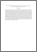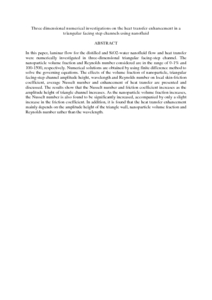# Three dimensional numerical investigations on the heat transfer enhancement in a triangular facing step channels using nanofluid

## Citation

Mohammed, K. A. and Abu Talib, A. R. and Abdul Aziz, N. and Ahmed, K. A. (2016) Three dimensional numerical investigations on the heat transfer enhancement in a triangular facing step channels using nanofluid. IOP Conference Series: Materials Science and Engineering, 152 (1). pp. 1-9. ISSN 1757-8981

## Abstract

In this paper, laminar flow for the distilled and SiO2-water nanofluid flow and heat transfer were numerically investigated in three-dimensional triangular facing-step channel. The nanoparticle volume fraction and Reynolds number considered are in the range of 0-1% and 100-1500, respectively. Numerical solutions are obtained by using finite difference method to solve the governing equations. The effects of the volume fraction of nanoparticle, triangular facing-step channel amplitude height, wavelength and Reynolds number on local skin-friction coefficient, average Nusselt number and enhancement of heat transfer are presented and discussed. The results show that the Nusselt number and friction coefficient increases as the amplitude height of triangle channel increases. As the nanoparticle volume fraction increases, the Nusselt number is also found to be significantly increased, accompanied by only a slight increase in the friction coefficient. In addition, it is found that the heat transfer enhancement mainly depends on the amplitude height of the triangle wall, nanoparticle volume fraction and Reynolds number rather than the wavelength.Preview
PDF (Abstract)
Three dimensional numerical investigations on the heat transfer enhancement in a triangular facing step channels using nanofluid.pdfView Item